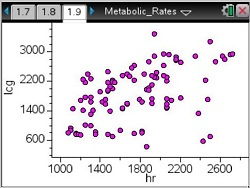# Activities

••• ##### Subject Area

• Math: Statistics: Data Analysis
• Math: Statistics: Probability and Random Variables

• ##### Author9-12

• ##### Device
•TI-Nspire™ CX/CX II
•TI-Nspire™ CX CAS/CX II CAS
• ##### Software

TI-Nspire™
TI-Nspire™ CAS

3.2

## Predicting Metabolic Rates of Astronauts#### Activity Overview

In this lesson, students will visually estimate correlation.

#### Objectives

In this lesson, students will:

• visually estimate correlations
• adjust weight in a weighted average to visually determine a curve of best fit for estimating metabolic rates
• find the sum of the squares of the difference between student estimates and NASA estimates of metabolic rates
• use the sums of squares to determine which students' estimates are closest to NASA's estimates